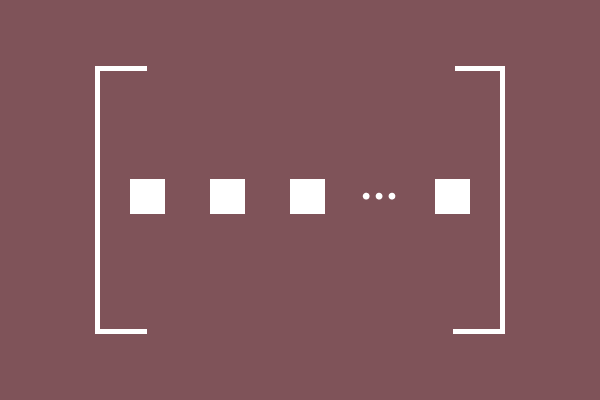# Row Matrix

A matrix that consists of all elements in only one row is called a row matrix.

## Introduction

A row matrix is one type of matrix. In this matrix, the elements are arranged in only one row and a number of columns. Hence, it is called a row matrix and also called as a row vector.For example, we have some elements and all elements are arranged in only one row. For separating all elements, we arrange them in different columns. In this case, let’s assume all elements are arranged in $n$ columns. Therefore, the elements are arranged in $1$ row and $n$ columns.

A matrix $M$ of the order $1 \times n$ is formed and it can be written in the following mathematical form.

$M$ $\,=\,$
${\begin{bmatrix} e_{11} & e_{12} & e_{13} & \cdots & e_{1n}\\ \end{bmatrix}}_1 \times n}$

The arrangement of elements in the matrix represents a rectangle shape. Hence, a row matrix is basically a rectangular matrix and it can be expressed simply as follows.

$M = {\begin{bmatrix} e_ij} \end{bmatrix}}_m \times n}$

In the case of a row vector, the number of rows $i = 1$. So, $m = 1$ but $j = n$. Hence, the simple form of a row matrix can be written in the following matrix form.

$M = {\begin{bmatrix} e_1j} \end{bmatrix}}_1 \times n}$

### Examples

The following matrices are best examples for a row matrix.

$(1).\,\,\,\,$ $A = \begin{bmatrix} 5 \end{bmatrix}$

$A$ is a row matrix of the order $1 \times 1$. Only one element is arranged in one row and one column in this matrix.

$(2).\,\,\,\,$ $B = \begin{bmatrix} -1 & 4 \end{bmatrix}$

$B$ is a row matrix of the order $1 \times 2$. In this matrix, two elements are arranged in one row but in two columns.

$(3).\,\,\,\,$ $C = \begin{bmatrix} 9 & 4 & 3 \end{bmatrix}$

$C$ is a row matrix of the order $1 \times 3$. Three elements are arranged in one row but in three columns in this matrix.

$(4).\,\,\,\,$ $D = \begin{bmatrix} 2 & 6 & 7 & 3 \end{bmatrix}$

$D$ is a row matrix of the order $1 \times 4$. In this matrix, four elements are arranged in one row but in four columns.

In all above four examples, the elements are arranged in only one row but the number of columns are different. Similarly, the arrangement of elements in all matrices forms a rectangle shape. So, a row matrix is always a rectangular matrix.

Latest Math Topics
Jun 26, 2023
Jun 23, 2023

###### Math Questions

The math problems with solutions to learn how to solve a problem.

Learn solutions

Practice now

###### Math Videos

The math videos tutorials with visual graphics to learn every concept.

Watch now

###### Subscribe us

Get the latest math updates from the Math Doubts by subscribing us.